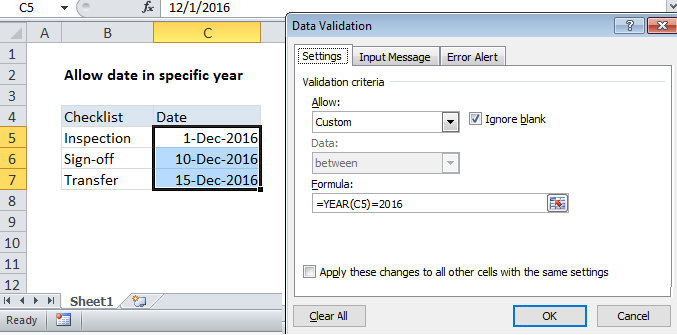## Excel Office

Excel How Tos, Tutorials, Tips & Tricks, Shortcuts

# Excel Data validation date in specific year

Using the example below, this tutorial shows how to create  Data validation date in specific year in Excel.

## Formula

`=YEAR(A1)=2016`

##Explanation

Note: Excel has several built-in data validation rules for dates. This page explains how to create a your own validation rule based on a custom formula in case you want or need more control and flexibility.

To allow a user to enter only dates in a certain year, you can use data validation with a custom formula based on the YEAR function.

Worked Example:   Count dates in current month in Excel

In the example shown, the data validation applied to C5:C7 is:

`=YEAR(C5)=2016`

### How this formula works

Data validation rules are triggered when a user adds or changes a cell value.

This custom validation formula simply checks the year of any date against a hard-coded year value using the YEAR function.

Worked Example:   How to calculate next scheduled event in Excel

When a user enters a value, the YEAR function extracts and compares the year to 2016:

`=YEAR(C5)=2016`

When the years match, the expression returns TRUE and validation succeeds. If the years don’t match, or if the YEAR function is not able to extract a year, validation fails.

### Date this year

To allow only dates in the current year, you can adjust the formula like this:

`=YEAR(C5)=YEAR(TODAY())`

The TODAY function will return the current date on an on-going basis, so formula returns TRUE only when a date is in the current year.

Worked Example:   Calculate days remaining in Excel

Note:  Cell references in data validation formulas are relative to the upper left cell in the range selected when the validation rule is defined, in this case C5.